Electron. J. Differential Equations, Vol. 2021 (2021), No. 92, pp. 1-20.

### Lower order for meromorphic solutions to linear delay-differential equations Rachid Bellaama, Benharrat Belaidi

Abstract:
In this article, we study the order of growth for solutions of the non-homogeneous linear delay-differential equation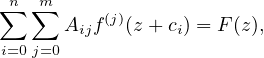where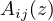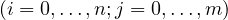,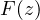are entire or meromorphic functions and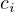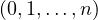are non-zero distinct complex numbers. Under the condition that there exists one coefficient having the maximal lower order, or having the maximal lower type, strictly greater than the order, or the type, of the other coefficients, we obtain estimates of the lower bound of the order of meromorphic solutions of the above equation.

Submitted June 25, 2021. Published November 18, 2021.
Math Subject Classifications: 30D35, 39B32, 39A10.
Key Words: Linear difference equation; linear delay-differential equation; meromorphic solution; order; type; lower order, lower type.

Show me the PDF file (356 KB), TEX file for this article.Rachid Bellaama Department of Mathematics Laboratory of Pure and Applied Mathematics University of Mostaganem, Algeria email: rachidbellaama10@gmail.com Benharrat Belaïdi Department of Mathematics Laboratory of Pure and Applied Mathematics University of Mostaganem, Algeria email: benharrat.belaidi@univ-mosta.dz